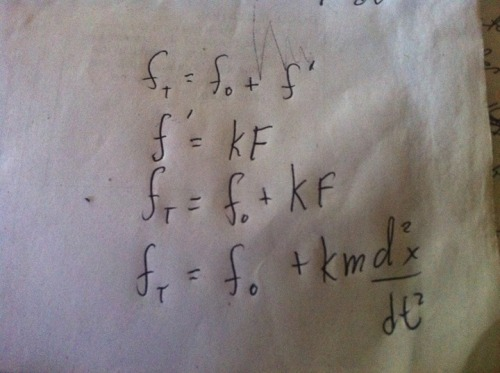# Non-robot related, physics question

Hello robodudes!!! I've got a question, it's not for a school homework(I promise :| ), it's for something that I'm currently working on, I just can't find any other equations anywhere that describes what I needed, so I've made my own....and I dunno if it's correct.

I was looking for an equation for a simple harmonic oscillator that will oscillate continuously untill a force(from the outside) is applied to it, transforming it as a driven(forced) harmonic oscillator that will have a frequency(and not amplitude{i.e displacement}, the amplitude should be always constant, with any force applied, even at the oscillator's natural state...every equation I can find has a changing amplitude due to the force{amplictude is dependent to the force applied}) dependent on the force applied, when the force is applied, the total frequency should get bigger, and after the force is applied, the total frequency will be dampen(i.e the magnitude of the frequency gets weaker), till it goes back to it's original frequency where it would oscillate continuously as a simple harmonic oscillator again, and the process will repeat if one applies another force...here's the equation I've made(note; the last one is the final equation describing what I wanted):here are the variables:

f(t)=total frequency

f(0)=natural frequency

f'=frequency added after the force is applied

k=spring constant of the oscillator

F=force applied

m=mass of where the force came from

d^2x/dt^2= acceleration(and immediate deceleration) of the frequency(i.e the oscillation) after the force was applied, total frequency will change over time after the force is applied, untill it reaches back to it's natural frequency, when it gets back to it's natural frequency, d^2x/dt^2 would be zero)

so, here's the summary:

an oscillator at it's natural state, will continuously oscillate forever with a natural frequency...... a force from the outside is applied to the oscillator.........the oscillator will then have a new frequency which is faster than it's natural frequency due to the force applied...... the amplitude will never change(constant, at any given force, it would also have the same amplitude at it's natural state)......the new frequency would then decelerate untill it reaches back to it's natural frequency....when it reached the natural velocity of it's frequency, the oscillator will be in it's natural state again.

is the formula correct?

cheers :)

f’ = kF?

f’ = kF ?

I think there’s some dimensional problem.

L.H.S dimension: [T^-1]

R.H.S dimension: [MLT^-2][L^-1][MLT^-2] = [M^2 L T^-4]

Dimensions don’t match, so it’s not possible.

I have assumed the spring constant is the force required to produce unit elongation…

Oh I got it!
I think, it’s just simple
f(t)=f(0)+df’/dt

Since in plank relationship;
E=hf…f’=E/h

And from the energy momentum equivalence;
f’=E/h=pc/h

the applied force as an energy/momentum acting in the oscillator to change it’s frequency, which accelerates it and then decelerates.
Is that correct?

Where is MarkusB when you

Where is MarkusB when you need himSorry, i do not have an answer to your problem, but I am sure that Markus, our LMR mathematician can helpEquation of a SHM is like

Equation of a SHM is like this (differential one)

d^2x/dt^2 + w^2x =0; This is for the undamped simple harmonic oscillator

You are trying to modify the frequency, which should deal with the “w” part, which is the angular frequency.

it MAY be written like this with boundary conditions-

d^2x/dt^2 + (w0+ (wf (t0-t) ) )^2 x =0 // this is when the time t is less than t0=time taken by the oscillator to go back to its Equilibrium state.(wf= angular frequency of the applied force,since it must be periodic.)

and

d^2x/dt^2 + (w0+ (wf (0) ) )^2 x =0 //when t>=t0;

I just guessed this…

Thanks mate!!
But can I use the equation that I’ve given below though?(on the “I got it comment”)